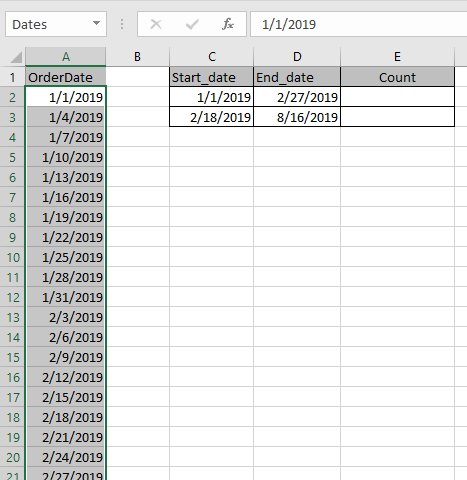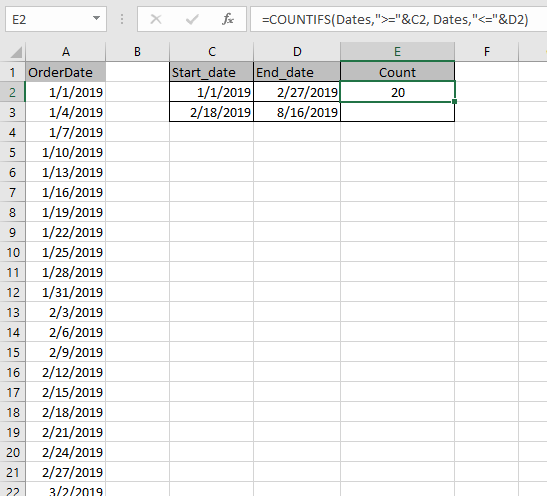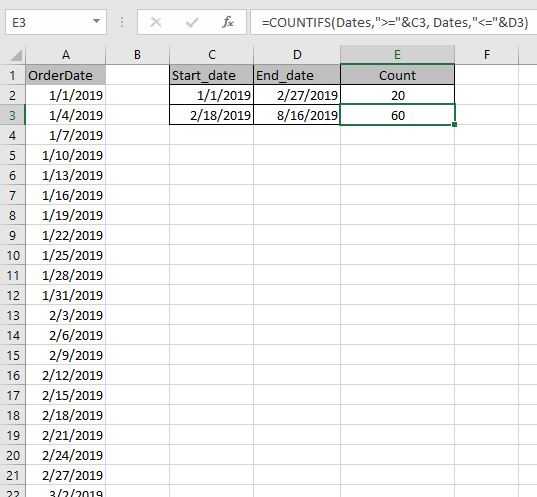# How to Count cells between dates in Excel

In this article, we will learn about how to Count cells between dates using COUNTIFS in Excel.

The COUNTIFS function gets the count dates that meet multiple criteria.
Syntax:

=COUNTIFS(Range ,">=" & date1, Range, "<=" & date2)

Range : Set of values
& : operator used to concatenate other operator.
Let’s understand this function using it an example.Here we have a list of dates from A2 : A245 named “Dates”.
We need to find count the fields which matches dates between the dates.

Use the Formula in E2 cell.

=COUNTIFS(Dates, ">=" & C2, Dates, "<=" & D2)As you can see the formula to find the dates between the dates worked and it gives the output 20. That means there are 20 cells which have dates between 1/1/2019 & 2/27/2019.

Now the formula for E3 cell.

=COUNTIFS(Dates,">="&C3, Dates,"<="&D3)The formula returns 60. So,there are 60 dates between 2/18/2019 & 8/16/2019.

Hope you understood how to Count cells between dates in Excel. Explore more articles on Excel COUNT function here. Please feel free to state your query or feedback for the above article.

Related Articles:

How to use the DAYS function in Excel

How to Count Days in Month in Excel

Calculate days,months and years in Excel

Calculate days, years and months from certain date in Excel

Popular Articles

50 Excel Shortcut to Increase Your Productivity : Get faster at your task. These 50 shortcuts will make you work even faster on Excel.

How to use the VLOOKUP Function in Excel : This is one of the most used and popular functions of excel that is used to lookup value from different ranges and sheets.

How to use the COUNTIF function in Excel : Count values with conditions using this amazing function. You don't need to filter your data to count specific values. Countif function is essential to prepare your dashboard.

How to use the SUMIF Function in Excel : This is another dashboard essential function. This helps you sum up values on specific conditions.

Terms and Conditions of use

The applications/code on this site are distributed as is and without warranties or liability. In no event shall the owner of the copyrights, or the authors of the applications/code be liable for any loss of profit, any problems or any damage resulting from the use or evaluation of the applications/code.